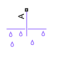# Reservoir (MA)

Boundary conditions for moist air network at constant pressure, temperature, moisture, and trace gas levels

• Library:
• Simscape / Foundation Library / Moist Air / Elements

•## Description

The Reservoir (MA) block sets boundary conditions in a moist air network. The volume of moist air inside the reservoir is assumed infinite. Therefore, the flow is assumed quasi-steady. Moist air leaves the reservoir at the reservoir pressure, temperature, specific humidity, and trace gas mass fraction. Moist air enters the reservoir at the reservoir pressure, but the temperature, specific humidity, and trace gas mass fraction are determined by the moist air network upstream.

You specify the reservoir pressure, temperature, amount of moisture, and amount of trace gas by entering block parameter values. Block parameters related to trace gas are ignored if Trace gas model in the Moist Air Properties (MA) block is set to `None`.

You can specify moisture as one of:

• Relative humidity, φw

• Specific humidity, xw

• Water vapor mole fraction, yw

• Humidity ratio, rw

You can specify trace gas as one of:

• Trace gas mass fraction, xg

• Trace gas mole fraction, yg

These moisture and trace gas quantities are related to each other as follows:

`$\begin{array}{l}{\phi }_{w}=\frac{{y}_{w}p}{{p}_{ws}}\\ {y}_{w}=\frac{{x}_{w}{R}_{w}}{R}\\ {r}_{w}=\frac{{x}_{w}}{1-{x}_{w}}\\ {y}_{g}=\frac{{x}_{g}{R}_{g}}{R}\\ {x}_{a}+{x}_{w}+{x}_{g}=1\\ R={x}_{a}{R}_{a}+{x}_{w}{R}_{w}+{x}_{g}{R}_{g}\end{array}$`

where:

• p is pressure.

• R is specific gas constant.

Subscripts `a`, `w`, and `g` indicate the properties of dry air, water vapor, and trace gas, respectively. Subscript `ws` indicates water vapor at saturation.

## Ports

### Conserving

expand all

Moist air conserving port associated with the reservoir inlet.

## Parameters

expand all

Select a specification method for the reservoir pressure:

• `Atmospheric pressure` — Use the atmospheric pressure, specified by the Moist Air Properties (MA) block connected to the circuit.

• `Specified pressure` — Specify a value by using the Reservoir pressure parameter.

Enter the desired pressure in the reservoir. This pressure remains constant during simulation.

#### Dependencies

Enabled when the Reservoir pressure specification parameter is set to ```Specified pressure```.

Select a specification method for the reservoir temperature:

• `Atmospheric temperature` — Use the atmospheric temperature, specified by the Moist Air Properties (MA) block connected to the circuit.

• `Specified temperature` — Specify a value by using the Reservoir temperature parameter.

Enter the desired temperature in the reservoir. This temperature remains constant during simulation.

#### Dependencies

Enabled when the Reservoir temperature specification parameter is set to ```Specified temperature```.

Select a moisture property:

• `Relative humidity` ― Specify moisture using the relative humidity.

• `Specific humidity` ― Specify moisture using the specific humidity.

• `Mole fraction` ― Specify moisture using the water vapor mole fraction.

• `Humidity ratio` ― Specify moisture using the humidity ratio.

Enter the desired relative humidity in the reservoir. This value remains constant during simulation.

#### Dependencies

Enabled when the Reservoir moisture specification parameter is set to `Relative humidity`.

Enter the desired specific humidity in the reservoir. This value remains constant during simulation.

#### Dependencies

Enabled when the Reservoir moisture specification parameter is set to `Specific humidity`.

Enter the desired water vapor mole fraction in the reservoir. This value remains constant during simulation.

#### Dependencies

Enabled when the Reservoir moisture specification parameter is set to `Mole fraction`.

Enter the desired relative humidity in the reservoir. This value remains constant during simulation.

#### Dependencies

Enabled when the Reservoir moisture specification parameter is set to `Humidity ratio`.

Select a trace gas property:

• `Mass fraction` ― Specify the trace gas mass fraction.

• `Mole fraction` ― Specify the trace gas mole fraction.

Enter the desired trace gas mass fraction in the reservoir. This value remains constant during simulation.

#### Dependencies

Enabled when the Reservoir trace gas specification parameter is set to ```Mass fraction```.

Enter the desired trace gas mole fraction in the reservoir. This value remains constant during simulation.

#### Dependencies

Enabled when the Reservoir trace gas specification parameter is set to ```Mole fraction```.

Relative humidity above which condensation occurs. Amount of moisture in the reservoir must be less than saturation.

The cross-sectional area of the reservoir inlet.

## Version History

Introduced in R2018a